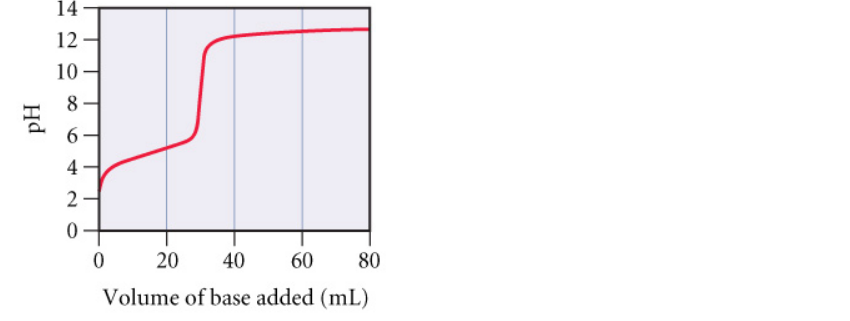# Problem: a. At what volume of added base is the pH calculated by working an equilibrium problem based on the initial concentration and Ka of the weak acid?b. At what volume of added base does pH = p Ka?c. At what volume of added base is the pH calculated by working an equilibrium problem based on the concentration and Kb of the conjugate base?

###### FREE Expert Solution
81% (432 ratings)
###### Problem Details

a. At what volume of added base is the pH calculated by working an equilibrium problem based on the initial concentration and Ka of the weak acid?

b. At what volume of added base does pH = p Ka?

c. At what volume of added base is the pH calculated by working an equilibrium problem based on the concentration and Kb of the conjugate base?What scientific concept do you need to know in order to solve this problem?

Our tutors have indicated that to solve this problem you will need to apply the Acid and Base Titration Curves concept. You can view video lessons to learn Acid and Base Titration Curves. Or if you need more Acid and Base Titration Curves practice, you can also practice Acid and Base Titration Curves practice problems.

What is the difficulty of this problem?

Our tutors rated the difficulty ofa. At what volume of added base is the pH calculated by work...as high difficulty.

How long does this problem take to solve?

Our expert Chemistry tutor, Dasha took 4 minutes and 46 seconds to solve this problem. You can follow their steps in the video explanation above.

What professor is this problem relevant for?

Based on our data, we think this problem is relevant for Professor Kimmel's class at RUTGERS.